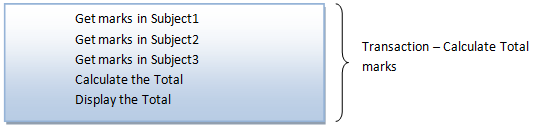# Database Transaction in DBMS

Suppose we have to calculate total marks of a student. Then what are the steps involved in it? Get the marks in each subject (say we have only 3 subjects), calculate the total and then display it. Here all the 3 steps are called as transactions. These are 3 small actions which cannot be further divided.From the above example, we understand that a transaction is a small piece of action / operation in the database. This piece of operations can be anything like insertion, deletion, updation or just retrieving the data from database. If the operation is retrieval then it does not modify the database. It pulls the required data from the database. If the operations are insert/ delete/ update, then it will modify DB. Then there exists two states for the transaction – before transaction and after transaction. Some transactions may include both retrieval and modification to DB.

Our example of calculating total marks above has only retrieval operation – it retrieves the marks from the database and performs summation to give it to user. It does not change the database. Suppose we have another transaction for updating CGPA of students. What happens in this case? Total marks of each student will be calculated as above, and then total marks will be compared with the CGPA marks criteria to decide the CGPA. Once CGPA is calculated, it will be updated into DB. Hence this transaction will have changes to DB. The state before transaction was without CGPA and after transaction is with CGPA. Here each step involved in calculating CGPA forms individual transaction.

Any operation or user request on DB is complete when the set of transactions involved in request is completed fully or not completed at all. It is not supposed to be in partial completion state. This is defined by transaction management concept below.

Translate »